# Matrix formulation of adjoint Kirchhoff datuming

Dimitri Bevc

Author has no known email address

# ABSTRACT

I present the matrix formulation of Kirchhoff wave equation datuming and show that upward and downward continuation are adjoint to each other. By expressing the datuming operator explicitly in matrix form, it is possible to examine the nature of the Hessian and to show that Kirchhoff datuming is not idempotent.

The Kirchhoff datuming codes I presented in earlier SEP reports (, ) are adjoint operators which pass the dot-product test; however, the adjoint formulation was arrived at by algorithmic considerations, not by formulating the operators in terms of matrix multiplications and taking their conjugate transpose. In this paper, I fill in this theoretical gap by presenting the matrix formulation of the datuming algorithm. I also show how Kirchhoff datuming can be cast as a multi-step depth extrapolation algorithm analogous to phase shift (, ) and finite difference () formulations presented in previous SEP reports.

I begin by briefly reviewing Kirchhoff datuming. I then present the matrix formulation of the operators, and conclude by incorporating these in a multi-step depth extrapolation formulation.

KIRCHHOFF DATUMING The upward continuation of a scalar wavefield can be expressed in integral form as:(1)
where P(0,z,t) is one trace of the upward continued wavefield at datum elevation z and lateral position x=0 (). The function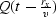is a time delayed filtered version of the input traces on the original datum. rx is the distance between input and output trace location and v is the propagation velocity. The purpose of the filtering operation is to compensate for the Hankel tail of the output wavelet.

For transformation of a wavefield from one datum to another, a discrete form of equation finallytext is a summation where each input trace Pi, recorded at location i along the lower datum is filtered and delayed by traveltime tij. Mathematically:(2)
Aij is an amplitude term incorporating spherical divergence and geometric terms,is the angle between the normal to the input surface and the straight line traveltime path connecting input location i and output location j, and tij is the traveltime between the input and output locations.

Equivalently, the sum can be performed in the frequency domain where each output trace is given by: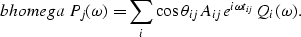(3)
By making the far-field approximation of the relevant Green's functions, the filtering operation used to compensate for the Hankel tail can be replaced by performing a half-order differential of the data traces (in 2-D). This is computationally attractive because the half-order differential is applied only once and does not depend on the indices i and j.

The algorithm can be applied to zero-offset data or to shot and receiver gathers. Prestack datuming is done by extrapolating the shots and the geophones separately (). The extension to three dimensions is straightforward and has the same algorithmic form. Equations finallytext through bhomega are for upward continuation. For downward continuation the adjoint process is used.

SINGLE OUTPUT TRACE Before writing down the matrix equations for Kirchhoff extrapolation one depth step at a time, I will present the matrix equations for the conventional formulation of Kirchhoff datuming: that is for extrapolation between two surfaces in one step.

Single output trace For illustration I consider the simple case of three input traces and one output trace. Equation bhomega can be expressed in matrix form as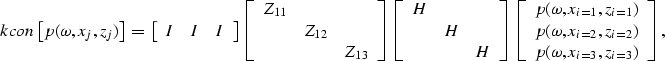(4)
where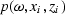is the Fourier transformed input wavefield recorded at datum zi andis an output trace at location (xj,zj). A half-order derivative is applied to the input data by the block matrices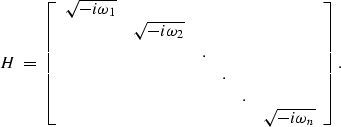(5)
If the calculation were performed in the time domain and the far-field approximation were not invoked, each of the H matrices would be replaced by a different matrix representing an i,j-variable convolution operator.

The block matrices Z11, Z12, and Z13 perform time shifts and amplitude scaling of individual data traces. For a given time shift tij, the time-shift matrix is given by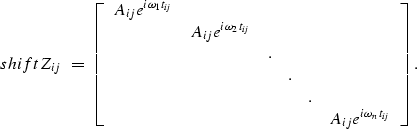(6)
The Aij factors are amplitude scaling terms which embody the effects of spherical divergence and obliquity.

Finally, the summation of the shifted input traces is represented by matrix multiplication with the row vector having block identity matrices I as its elements. The summation matrix and the Zij matrix can be combined to simplify equation kcon to:(7)

TIME-DOMAIN DATUMING AND MIGRATION For completeness, I show how the matrices differ when datuming is implemented in the time domain.

If Kirchhoff datuming is implemented in the time domain, the Hankel tail can be compensated for by performing an m-long i,j-dependent convolution. Then the matrix H becomes(8)

The time-shift tij is represented by a sparse matrix which is non-zero along one particular diagonal (or band) corresponding to the particular shift. For example, a time-shift matrix corresponding to a shift of two time samples is given by: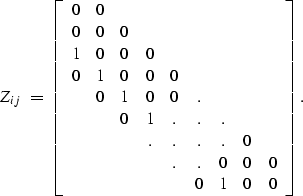(9)
If the data are linearly interpolated, the matrix will be banded (see for example Claerbout ).

Kirchhoff time migration Time migration by hyperbola summation is similar to Kirchhoff datuming except that in time migration, the traces are shifted by a time-variant amount given by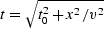.In order for equation kcon2 to represent time migration, the hyperbolic shifts would make the time-shift matrix look something like this: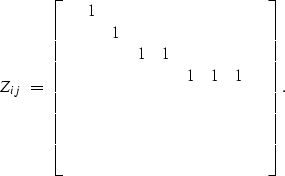(10)

MULTIPLE OUTPUT TRACES In this section I generalize equation kcon2 to multiple output traces. For illustrative purposes, I write down the matrix equation for three input traces and three output traces. The wavefield at the upper datum z is(11)
The time-shift matrices Zij correspond to equation shift with ij corresponding to the time shift between input and output locations i and j.

Equation  kmult represents the upward extrapolation of a wavefield between two arbitrarily shaped surfaces.

Downward continuation The downward continuation operator is derived by taking the complex conjugate transpose of the operator in equation kmult. This yields the adjoint operator: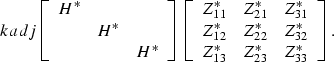(12)

Equation kadj is the downward continuation operator for the extrapolation of a wavefield between two arbitrarily shaped surfaces.

IDEMPOTENCE AND THE HESSIAN

Downward continuation followed by upward continuation is given by the multiplication of the operators in equations kmult and  kadj:(13)

If the datuming is performed between level datums with constant velocity, the matrix equations can be simplified by making the following observations and substitutions: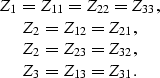Performing the indicated substitutions, yields the simplified matrices: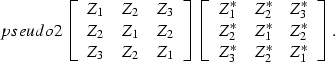(14)

Using the notation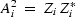and carrying out the multiplication we obtain the following expression for the Hessian matrix:(15)

This banded matrix is diagonally dominant with real valued terms on the main diagonal and complex terms along the other diagonals. The datuming operator is not idempotent because of the non-zero terms off the main diagonal. The consequence of this is that if the Kirchhoff datuming operator and its adjoint are applied repeatedly, energy will be lost with each application.

STEP-BY-STEP WAVEFIELD EXTRAPOLATION

The operator in equation kmult can be used to extrapolate a wavefield between two horizontal planes. In this case, each application of the extrapolation algorithm is expressed by the matrix operator Wi which is the operator in equation kmult. For three depth levels, the Kirchhoff datuming algorithm can be formulated as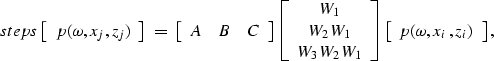(16)
The algorithm can be generalized for any number of depth levels and any datum geometry. A, B, and C are geometry matrices which correspond to the datum shape. These matrices represent the action of selecting data traces on the new datum. The matrices A, B, and C have non-zero elements only at certain locations along their diagonals where the wavefield is to be extracted. For a more detailed description of these matrices, see Popovici .

For downward continuation, the conjugate-transpose of equation steps is taken to obtain the adjoint process:(17)
whereis data recorded at some irregular datum. The matrices W*i correspond to the operator in equation kadj.

DISCUSSION AND CONCLUSIONS For real problems, the operator representations of the preceding section result in huge, albeit sparse, matrices. In the actual computer implementation of Kirchhoff datuming these huge matrix operators are not actually stored. The numerical algorithms apply these linear operators indirectly and much more efficiently.

Equations kmult and kadj represent upward and downward continuation of a scalar wavefield in one step. These are the matrix equivalents of the frequency domain datuming implementation I have presented in previous papers  (, ). A corresponding representation of Berryhill's time domain algorithm can be obtained by changing the H matrices to convolutional operators and casting the rest of the matrices in equations kmult and kadj in the time domain (, ).

Kirchhoff datuming is represented as extrapolation of one depth level at a time in equations steps and stepadj. While computationally less efficient than the one-step method of equations kmult and kadj, it allows greater flexibility in specifying the velocity model. With some approximations, the wavefield can be extrapolated through a v(x,y,z) velocity model. The multi-step operator results in steeper Kirchhoff summation trajectories for each step than the one-step method. This may allow steeper events to be handled with less aperture, therefore gaining some computational advantage.

By examining the simplified datuming operator for the special case of flat datums and constant velocity, I observe that the datuming operator is not idempotent and that the Hessian is diagonally banded.

ACKNOWLEDGEMENTS I am grateful to Stew Levin for critically reviewing this paper and to Jun Ji, Dave Nichols and Mihai Popovici for enlightening discussions.

[SEP,GEOTLE,MISC]